# Free Graphing Calculator 2

## A powerful flexible graphing calculator . . . and it's free!

3.94 EDUCATION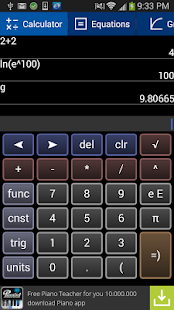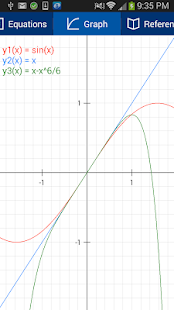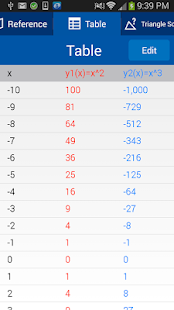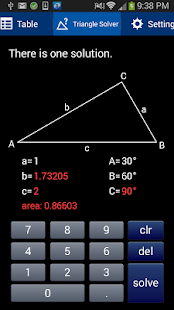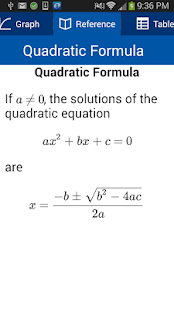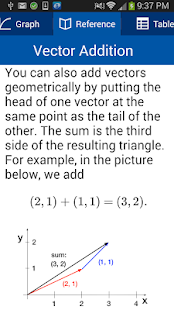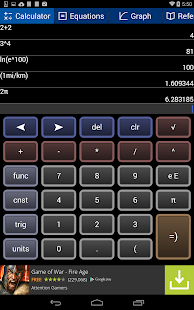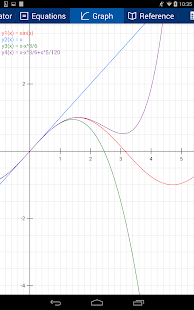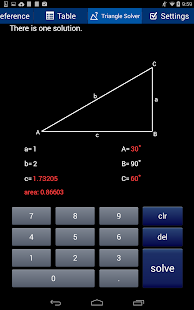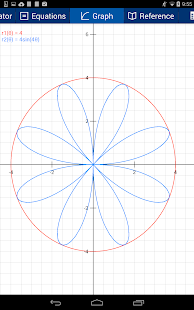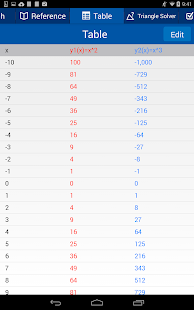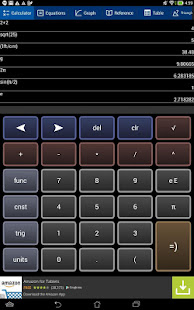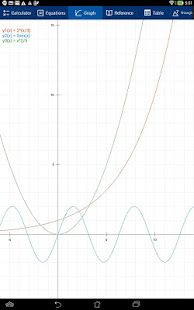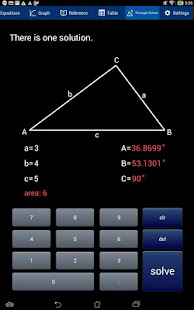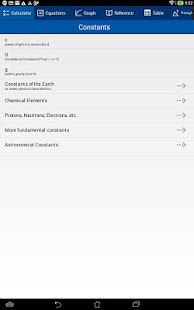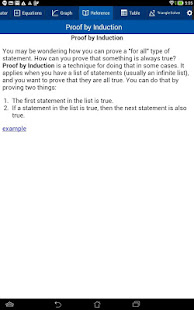A powerful, flexible graphing calculator . . . and it's free!

Does far more than most of the paid calculators out there . . . let alone the free ones.

Features:

1) Scientific Calculator. Simple to grasp and easy to use, but powerful features are available when you need them. Available functions include the following:

• the usual arithmetic functions and exponentiation.
• square root, cube root, nth root, natural log, log base 10, log of arbitrary base, absolute value, factorial, permutations (nPr), combinations (nCr), modulus, random integer, bell curve, cumulative normal distribution, decimal to fraction.

2) Graphing. Capabilities:

• Graph up to four equations at once.
• Graphs are labeled.
• You can drag the graph or pinch to zoom in or out.
• Calculator can find roots and intersections.
• Graph in polar coordinates.
• Graph parametric equations
• Can graph implicit functions, such as x^2+y^2-4=0. Most calculator apps can't do this!

3) A unit converter. With a tap, you can enter the result of your conversion into the calculator. Currently converts different units of the following: acceleration, angle, area, density, distance, energy, force, mass, power, pressure, speed, temperature, time, and volume. Great for doing physics homework!

4) Constants for scientific calculations -- speed of light, strength of gravity at Earth's surface, etc. etc. etc. Tapping on a constant will insert it into your calculation -- i.e, you don't have to key in the value. Again, great for doing physics homework!

5) It can make a table of the values of a function you enter. You can choose the starting x value of the table, as well as how much x increases for each successive row.

6) Help screens linked directly to many of the available functions and constants. Tap the disclosure arrow to see the definition.

7) Forgot the quadratic formula? Or the double-angle formulas for sine and cosine? The math/science reference hits the high points of various subjects. Currently includes algebra, differential and integral calculus, geometry, trigonometry, vectors, vector calculus, and classical mechanics.

8) Statistics -- enter data and make a histogram, box and whisker plot, or scatter plot with optional regression line. Also statistical distributions (under functions > statistics) -- beta, Cauchy, chi, chi squared, continuous uniform, Erlang, exponential, Fisher-Snedecor, gamma, inverse gamma, Laplace, log-normal, normal, Pareto, Rayleigh, Student's T, and Weibull.

I'd love to hear your comments or suggestions. Because of spammers, my email address is not in this description, but it is inside the app, and it's great to hear from real users.

Attempted to fix launch issue where one has to switch tabs to get it to work

### Details and Recent Ratings

Size: 0 MB
Version: 10.4 by William Jockusch
Updated: 03 March 2020 (1301 days ago)
Released: 19 August 2014
Installations: more than 100 000
5 Stars: 635
4 Stars: 178
3 Stars: 65
2 Stars: 65
1 Star: 165

### Similar apps

Android Game Categories
Android App Categories